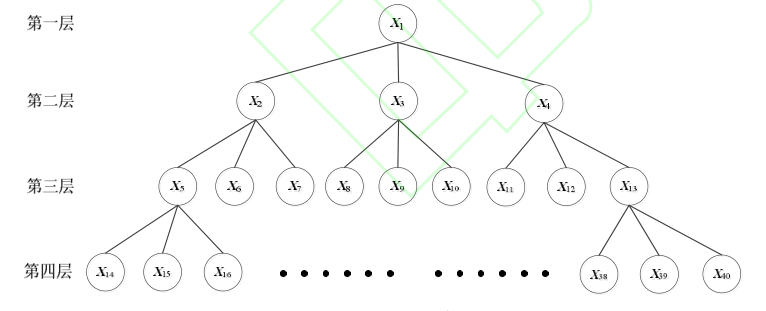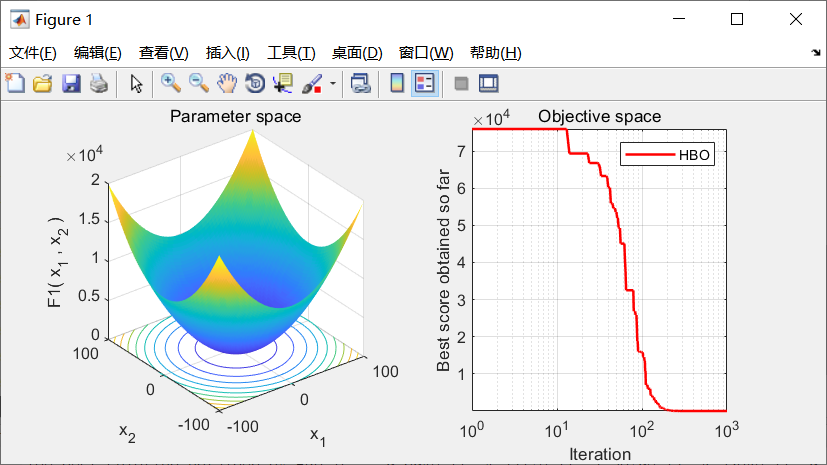• 堆优化
千次阅读
2022-03-16 07:47:21

堆优化的Dijkstra算法

迪杰斯特拉算法(Dijkstra)是由荷兰计算机科学家狄克斯特拉于1959 年提出的，因此又叫狄克斯特拉算法。是从一个顶点到其余各顶点的最短路径算法，解决的是有权图中最短路径问题。迪杰斯特拉算法主要特点是从起始点开始，采用贪心算法策略，每次遍历到始点距离最近且未访问过的顶点的邻接节点，直到扩展到终点为止。

这里的Dijkstra算法是经过堆优化的算法，用于解决稀疏图问题。

基本思想是通过邻接表储存每个点所能到达的点。用一个dist[]数组来储存初始点到达每个节点的最短距离，并初始化为无穷大。首先将初始点推入队列中，然后通过这个点来遍历每一个子节点并将每个使得初始节点到当前点的距离加上这个点到每个子节点的距离小于当前dist[]的值替换掉，并推入队列中，由于这里是小根堆，所取到的队首的值一定是当初始节点距离的点并开始下一轮遍历，以此类推直至找到目标节点的最短距离。

代码：

#include<iostream>
#include<cstring>
#include<algorithm>
#include<queue>
using namespace std;
typedef pair<int,int> PII;
const int N=200010;
int ne[N],h[N],e[N],idx;//邻接表储存方式
int n,m;
int w[N],dist[N];//w储存每条边的权值，dist储存初始节点到每一个节点的最短距离
bool st[N];//储存每个点是否找到了最短距离
priority_queue<PII,vector<PII>,greater<PII>> heap;//小根堆的定义
void insert(int a,int b,int c)
{
e[idx]=b;
w[idx]=c;//储存权值
ne[idx]=h[a];
h[a]=idx++;
}
int dijkstra()
{
memset(dist,0x3f,sizeof dist);//将距离初始初始化为正无穷
heap.push({0,1});//将初始节点推入队列中
while(!heap.empty())
{
auto t=heap.top();
heap.pop();
int cnt=t.second,distance=t.first;//t.first代表当前节点到初始节点的最短距离，second代表当前是哪一个节点
if(st[cnt]) continue;
st[cnt]=true;
for(int i=h[cnt];i!=-1;i=ne[i])//遍历这个链表
{
int j=e[i];
if(dist[j]>distance+w[i])//如果当前节点到子节点的距离加上这条边的权值小于之前头结点到子节点的距离
{
dist[j]=distance+w[i];
heap.push({dist[j],j});
}
}
}
if(dist[n]!=0x3f3f3f3f) return dist[n];
return -1;
}
int main()
{
scanf("%d%d",&n,&m);
memset(h,-1,sizeof h);
while(m--)
{
int a,b,c;
scanf("%d%d%d",&a,&b,&c);
insert(a,b,c);
}
printf("%d",dijkstra());
return 0;
}算法 数据结构 队列 分布式
更多相关内容
• 堆优化dijkstra算法。使用邻接表。邻接表的应用案例。 Dijkstra算法是典型的单源最短路径算法，用于计算一个节点到其他所有节点的最短路径。主要特点是以起始点为中心向外层层扩展，直到扩展到终点为止。Dijkstra...c++ 最短路
• dijkstra时间优化，堆优化，优先队列，最短路算法，O（NlogN）空间时间优化，链式存储，邻接表存图，NOIP，ACM算法竞赛，数据结构最短路
• 这是实现最短路径求法dijstra 算法的代码。并且对该算法进行了堆优化，具有更快的运算速度。
• ## prim算法堆优化

千次阅读 2020-12-10 16:53:40
首先，prim算法的思想是，从一个起点...如果没有进行堆优化，那从这个数组里找出最小距离的点的时间复杂度是O（n）,通过堆优化，我们获取最小值的时间复杂度则为O（log2n）,在图的点数目很大时，能有效的提升效率。 #in

首先，prim算法的思想是，从一个起点开始，通过不断的纳入与当前生成树距离最短的且不在生成树中的点来构造最小生成树，当所有点都纳入到生成树以后，就得到了最小生成树。使用邻接表来存储图，能使得所需的空间最小。通过一个数组来存放各个点到当前生成树的最短距离，可以避免重复比较，从而提升效率。但我们所要获取的是该数组里的距离最小的那个点。如果没有进行堆优化，那从这个数组里找出最小距离的点的时间复杂度是O（n）,通过堆优化，我们获取最小值的时间复杂度则为O（log2n）,在图的点数目很大时，能有效的提升效率。

#include<iostream>
using namespace std;

struct VTshortest {
int Tdot;       //到生成树的哪个点距离最短
int distance;    //到生成树的最短距离
};

int destination;    //终点
int distance;       //距离
};
struct result {
int orgin;
int destination;
};

void  heapify_up(VTshortest* shortest,int* heap,int* map,int location) {     //因为值减小，引起堆被破坏，所要进行的操作，值变小元素上浮
if (map[location] == 0) {
return;
}
if (shortest[heap[(map[location] - 1) / 2]].distance > shortest[heap[map[location]]].distance) {
int x = heap[(map[location] - 1) / 2];
swap(heap[(map[location] - 1) / 2], heap[map[location]]);
swap(map[location], map[x]);
heapify_up(shortest, heap, map, location);
}
}

void  heapify_down(VTshortest* shortest, int* heap, int* map, int top, int heap_end) {  //因为堆顶和堆尾互换，引起的堆被破坏，所要进行的操作,值大元素下沉
if (top*2+1 > heap_end && top*2+2 > heap_end) {
return;
}
if (top * 2 + 1 <= heap_end && top * 2 + 2 <= heap_end) {
if (shortest[heap[top * 2 + 1]].distance < shortest[heap[top * 2 + 2]].distance) {
if (shortest[heap[top * 2 + 1]].distance < shortest[heap[top]].distance) {
int x = heap[top * 2 + 1];
int y = heap[top];
swap(heap[top], heap[top * 2 + 1]);
swap(map[y], map[x]);
int top_lc = top * 2 + 1;
heapify_down(shortest, heap, map, top_lc, heap_end);
}
}
else {
if (shortest[heap[top * 2 + 2]].distance < shortest[heap[top]].distance) {
int x = heap[top * 2 + 2];
int y = heap[top];
swap(heap[top], heap[top * 2 + 2]);
swap(map[y], map[x]);
int top_rc = top * 2 + 2;
heapify_down(shortest, heap, map, top_rc, heap_end);
}
}
}
if (top * 2 + 1 <= heap_end && top * 2 + 2 > heap_end) {
if (shortest[heap[top * 2 + 1]].distance < shortest[heap[top]].distance) {
int x = heap[top * 2 + 1];
int y = heap[top];
swap(heap[top], heap[top * 2 + 1]);
swap(map[y], map[x]);
return;
}
}
}

VTshortest* shortest = new VTshortest[num];   //用来存放，各个点到当前生成树的最短距离，不直接相连，距离无穷大（999），已经在生成树里的距离为0
for (int i = 0; i < num; i++) {
shortest[i].Tdot = -1;
shortest[i].distance = 999;               //999表示无穷大
}
int finish=0;                   //完成个数
int cDot = 0;                   //指向最近加入生成树的点
int* heap = new int[num];       //堆  ，堆里存的是shortest的下标，就是表示如果把shortest变成堆，那各个元素是处在堆的哪个位置
int heap_end=num-1;             //堆尾部
int* map = new int[num];        //map是shortest到heap的映射，map的下标对应是哪个shortest的下标（元素），map[下标]对应的是该shortest在堆的下标
result* re = new result[num];   //存放边结果
for (int i = 0; i < num; i++) {  //初始化堆和shortest与堆的对应关系
map[i] = i;
heap[i] = i;
}
while (finish!=num) {
cDot = heap;             //取堆顶，即与当前生成树的距离最短的点
cout<<"堆顶元素：" << heap << endl;
int x = heap;
int y = heap[heap_end];
swap(heap, heap[heap_end]);     //取完，说明该点已经完成，调整到堆尾，堆长度减一
swap(map[x], map[y]);
heap_end--;
heapify_down(shortest, heap, map, 0, heap_end);
cout << "当前堆情况：";
for (int i = 0; i <= heap_end; i++) {
cout << heap[i] <<"("<<shortest[heap[i]].distance<<")"<< "  ";
}
cout << endl;
//记录结果
re[finish].orgin = shortest[cDot].Tdot;
re[finish].destination = cDot;
finish++;

shortest[cDot].distance = 0;
while (temp != NULL) {
if (temp->distance < shortest[temp->destination].distance) {
shortest[temp->destination].distance = temp->distance;
shortest[temp->destination].Tdot = cDot;
heapify_up(shortest, heap, map, temp->destination);
cout << "当前堆情况：";
for (int i = 0; i <= heap_end; i++) {
cout << heap[i] << "(" << shortest[heap[i]].distance << ")" << "  ";
}
cout << endl;
}
temp = temp->next;
}
}
for (int i = 0; i < num ; i++) {
cout << "(" << re[i].orgin << "," << re[i].destination << ")   ";
}
}

展开全文算法 数据结构
• 智能优化算法：堆优化算法 文章目录智能优化算法：堆优化算法1.算法原理2.算法结果3.参考文献4.Matlab代码 摘要：堆优化算法(Heap-based optimizer，HBO)是 Askari 等人在 2020 年提出的一种新型智能优化算法。它...

# 智能优化算法：堆优化算法

### 文章目录

摘要：堆优化算法(Heap-based optimizer，HBO)是 Askari 等人在 2020 年提出的一种新型智能优化算法。它利用堆结构模拟了公司的层级结构，采用了堆的概念形成个体之间的交互，并且构建了三种构造新解的数学模型。具有收敛速度快，精度高的特点。

## 1.算法原理

HBO 模拟公司层次结构建立的树状结构，目前它选择的是三元堆或者说是一个三叉树，具体详见图 1。企业等级制度的最终目标是以最好的方式完成与业务相关的任务，主要包括三个数学模型:下属与直接领导的交互、与同事的交互和个体的自我贡献。图1.三元堆

图1中 X 1 X_1 所在的层次为最高层第一层，仅有一个个体(其适应度值最高，为最优个体)，； X 2 ∼ X 4 X_2\sim X_4 所在的层次为第二层，3 个个体(它们的适应度值低于第一层个体的适应度值，以下类似)； X 5 ∼ X 13 X_5\sim X_{13} 所在的层次为第三层，9 个个体；如此，第四层应该有 27 个体；所有这些个体组成一个群体，其种群大小为 40。其中，第一层至第三层在本文中称为高层，第四层为低层(其中个体的适应度值低于高层个体的适应度值)。从 X 2 X_2 开始所有的个体都是通过直接领导和同事的引导进行更新。以 X 8 X_8 为例，由于堆独特的结构，与 X 8 X_8 在同一层次的个体均为其同事，为 X 5   X 13 X_5 ~X_{13} ，且只有一个直接领导 X 3 X_3 。然而对于最高领导 X 1 X_1 ，它所在的层是最高层，没有直接领导，并且该层只有 X 1 X_1 一个个体，也不存在同事。

与直接领导交互的数学模型可以描述为:
X i j ( t + 1 ) = B j + γ λ ∣ B j − X i j ( t ) ∣ (1) X_i^j(t+1)=B^j+\gamma \lambda |B^j-X_i^j(t)|\tag{1}

γ = ∣ 2 − 4 ∗ m o d ( t , 25 ) / 25 ∣ (2) \gamma=|2-4*mod(t,25)/25| \tag{2}

λ = 2 r − 1 (3) \lambda = 2r-1 \tag{3}

其中， t t 是当前迭代次数， T T 是最大迭代次数， j j 是一个解向量的第 j j 个分量， B B 是当前个体的直接领导。 r r 是均匀分布在[0,1]中的随机数。在迭代过程中， γ γ 是一个三角波，它的值在 1 的左右波动，从 2 到 0，或从 0 到 2。

在堆中，位于同一层的个体都是其同事，每个个体 X i X_i 根据其随机选择的同事$S_r$ 更新其位置，其数学模型见式(4)。
X i j ( t + 1 ) = { S r j + γ λ ∣ S r j − X i j ( t ) ∣ , f ( S r ) < f ( X i ( t ) ) X i j + γ λ ∣ S r j − X i j ( t ) ∣ , e l s e (4) X_i^j(t+1)=\begin{cases} S_r^j+\gamma \lambda|S_r^j-X_i^j(t)|,f(S_r)<f(X_i(t))\\ X_i^j+\gamma \lambda|S_r^j-X_i^j(t)|,else \end{cases}\tag{4}
其中， f f 是个体的目标函数。对于最小极值问题，若 f ( S r ) < f ( X i ) f (S_r)<f(X_i) ，个体可以探索 S r S_r 周围的区域；若 f ( S r ) ≥ f ( X i ) f (S_r )≥ f (X_i ) ，个体可以探索 X i X_i 周围的区域，以保证搜索向好的方向发展。

在个体的自我贡献的模型中，个体在前一次迭代中的一些位置信息会一直保留到下一次迭代。即个体 X i X_i 在下一次迭代中不会改变其第 j j 个分量的值。
X i j ( t + 1 ) = X i j ( t ) (5) X_i^j(t+1)=X_i^j(t)\tag{5}
在 HBO 中， p 1 p_1 p 2 p_2 p 3 p_3 决定了个体将会在这三个数学模型中选择哪个模型进行更新。选择概率的计算方法如下:
p 1 = 1 − t / T (6) p_1=1-t/T \tag{6}

p 2 = p 1 + ( 1 − p 1 ) / 2 (7) p_2=p_1+(1-p_1)/2 \tag{7}

HBO 通过 p 1 p_1 选择自我贡献模型更新个体，通过 p 2 p_2 选择与直接领导交互的数学模型更新个体，通过 p 3 p_3 选择与同事交互的数学模型更新个体，其中 p 3 = 1 p_3 =1

算法 1: 堆优化算法
Step1: 设置参数并随机初始化种群
Step2: 评估种群中个体的适应度值，获取全局最优解
Step3: 构建堆
Step4: for t=1 to T do
Step5: for i= N to 2 do
Step6: for j=1 to D do
Step7: p=rand
Step8: if p≤p 1
Step9: 通过公式（5）更新个体位置
Step10: else if p > p 1 & p ≤ p 2
Step11: 通过公式（1）更新个体位置
Step12: else
Step13: if p > p 2 & p ≤ p 3
Step14: 通过公式（4）更新个体位置
Step15: end if
Step16: end if

Step17: end for
Step18: 边界控制，计算个体的适应度值
Step19: 贪心选择更新种群
Step20: 更新堆，更新全局最优解
Step21: end for
Step22: end for
Step23: 输出全局最优解

## 2.算法结果## 3.参考文献

Qamar Askari,Mehreen Saeed,Irfan Younas. Heap-based optimizer inspired by corporate rank hierarchy for global optimization[J]. Expert Systems With Applications,2020,161:

张新明,温少晨,刘尚旺.差分扰动的堆优化算法[J/OL].计算机应用:1-9[2021-12-11].http://kns.cnki.net/kcms/detail/51.1307.TP.20211014.1631.021.html.

## 4.Matlab代码

展开全文算法
• 迪杰斯特拉算法及其堆优化 迪杰斯特拉算法是一种求解图的单点最短路径的算法。 迪杰斯特拉算法的原理是 1.首先在没有中间节点的情况下，也就是直达路径中找到到达某点p的最短路径。易知，该路径一定是原点到点p的...

## 迪杰斯特拉算法及其堆优化

迪杰斯特拉算法是一种求解图的单点最短路径的算法。
一句话来说就是找最近点，更新相邻距离，再找最近点，更新相邻距离
迪杰斯特拉算法的原理是
1.首先在没有中间节点的情况下，也就是直达路径中找到到达某点p的最短路径。易知，该路径一定是原点到点p的最短路径。将点p标记在vis数组中，并将最短路径的值存在dist数组中。
2.再对所有节点进行松弛操作，也就是下一个节点的最短路径有两种情况，一种是经过某个已知的最短路（就是被vis标记的最短路径），第二种情况是直达。所以，求解下一个最短路径就是求解递推公式
dist[i]=min(dist[i] , map[i][now]+dist[now])
now是上一个最短路径。
3.找到最短的dist并标记在vis中，并迭代2，3步。
总体来说，迪杰斯特拉算法是按从小到大的顺序求解到各个点的最短路径，每求出一个最短路径，下次循环时就判断所有的点（其实等同于没有求出最短路径的点）如果经过上一个已经求出最短路径的点，是否会出现更短的路径（这一步就成为松弛操作）。在遍历所有点的同时，找到没有求出最短路径的点中的最短路径，并保存。
没有优化的迪杰斯特拉算法的时间复杂度为O(n*n)。
下面是一张图解：以下是参考代码：

#include<bits/stdc++.h>
using namespace std;
#define inf 0x7fffffff
long long int dist;
int Map;
int vis;
int main(){
int n,m,s;
while(~scanf("%d%d%d",&n,&m,&s)){
for( int i=1;i<=n;i++){
for( int j=1;j<=n;j++){
Map[i][j]=0x7fffffff;
}
dist[i]=0x7fffffff;
}
memset(vis,0,sizeof(vis));
for( int i=1;i<=m;i++){
int from,to,val;
cin>>from>>to>>val;
Map[from][to]=min(val,Map[from][to]);
Map[to][from]=min(val,Map[from][to]);
}
dist[s]=0;
vis[s]=1;
int start=s;
for( int j=1;j<=n;j++){
int next, minn=0x7fffffff;;
for( int i=1;i<=n;i++){
if(Map[start][i]!=inf) {
dist[i]=min(dist[i],Map[start][i]+dist[start]);
}
if(vis[i]==0&&dist[i]<minn){
next=i;
minn=dist[i];
}
}

if(minn==inf)break;
start=next;
vis[start]=0;
}
for( int i=1;i<=n;i++){
cout<<dist[i];
if(i!=n) cout<<" ";
}
cout<<endl;

}
}


对迪杰斯特拉算法的优化：
上面的迪杰斯特拉算法主要缺陷是，每当找到一个最短路径，如果需要找下一个最短路径，就需要在完成松弛操作之后，遍历dist数组，寻找其中的最小值。遍历dist数组的时间复杂度为O(n)。
如果图的边数为n*(n-1)，那么每找到一个最小值，所要进行的松弛操作数就是n-1，这和遍历dist数组可以同时进行，算法优化的空间不大。
然而，如果是稀疏图，每找到一个最小值，所要进行的松弛操作数就远小于n-1，这时就可以对算法进行优化。优化的关键是省去对dist的线性查找，如果每次可以直接返回dist中的最大值，就可以大大减小算法的时间复杂度。
堆优化后的迪杰斯特拉算法复杂度为ElogE

#include<bits/stdc++.h>
using namespace std;
#define inf 0x7fffffff
struct p{
long long int to,val,next;
};
p edge;
long long int dist;
int vis;
int cnt;
void add_edge( long long from,long long to,long long  val){
edge[++cnt].val=val;
edge[cnt].to=to;
}
struct node{
long long int from,val;
bool operator <(const node &a ) const{
return a.val<val;
}
};
int main(){
int n,m,s;
while(~scanf("%d%d%d",&n,&m,&s)){
cnt=0;
memset(edge,0,sizeof(edge));
for( int i=1;i<=n;i++){
dist[i]=1e12;
vis[i]=0;
}
for( int i=1;i<=m;i++) edge[i].val=1e12;
for( int i=1;i<=m;i++){
long long int from,to,val;
scanf("%lld%lld%lld",&from,&to,&val);
}
dist[s]=0;
priority_queue<node>q;
q.push({s,0});
while(!q.empty()){
node now=q.top();
q.pop();
if(vis[now.from]) continue;
vis[now.from]=1;
int j=edge[i].to;
if(dist[now.from]+edge[i].val<dist[j]){//进行松弛操作
dist[j]=dist[now.from]+edge[i].val;
q.push({j,dist[j]});
}
}
}
for( int i=1;i<=n;i++){
printf("%lld ",dist[i]);
}
cout<<endl;
}
}


迪杰斯特拉算法的优化可以总结为以下几步：
1.将最短距离出队。
2.进行松弛操作，并将成功松弛的点入队。

展开全文算法 数据结构 leetcode
•算法
• dijkstra算法及其堆优化 （个人笔记写给自己看的） 数据结构：链式前向星，优先队列 dijkstra算法: vis数组初始化为0，dis数组初始化为inf(很大的值如:0x7fffffff),设起点为s，则d[s]=0，vis[s]=1 for循环n次以下...
• } 3.dijkstra算法 步骤： ①写好链式前向星 ②dist和vis数组准备好 ③写好优先级队列 只适用于边权非负的图， 堆优化的dijkstra算法 priority_queue默认是一个大根堆，常用方法如下： [外链图片转存失败,源站可能有...
• 【智能优化算法-堆优化算法】基于堆优化算法求解单目标优化问题附代码（Heap-BasedOptimizer，HBO).zipmatlab
• Dijkstra——邻接表存储的堆优化算法 在明白原理后（上一篇讲过），发现朴素算法的时间复杂度太大，在找最短的距离需要枚举n个方案，但是在优先队列里面的小跟堆直接找堆顶就行。 优化思路： 用邻接表存图，然后用...算法 数据结构 c++
• Python解决最短路径问题—Dijkstra算法+堆优化最短路问题介绍Dijkstra算法介绍算法思想Dijkstra算法步骤代码 宇智波一打七今天学习了一个新算法，迫不及待和大家分享一下 。 最短路问题介绍 在一个赋权图中，从一个...python 算法
• 但是对于 稠密图 来说边数接近点数的平方个，如果稠密图使用堆优化版的Dijkstra算法，那么时间复杂度将会是 O( n2∗log nn^2*log\ nn2∗log n)，显然不如直接使用朴素Dijkstra算法。所以 堆优化版的Dijkstra算法 更...最短路 优先队列 C++
• 最短路：dijkstra堆优化（不开小根堆优化c++ c语言 算法
• ## dij最短路+堆优化

千次阅读 2018-11-24 23:34:41
堆优化dij采用堆这种数据结构优化出队顺序，因此时间复杂度较普通dij由n^2降低为nlogn*/ //算法时间复杂度O(n^2logn),空间复杂度O(m^2) #include using namespace std; const int maxn=1005; const int INF=0x3f3f3...
• ## Dijkstra算法及其堆优化代码详解

千次阅读 多人点赞 2019-04-29 19:36:23
补充：基于堆优化的dijkstra算法代码 可以把时间复杂度从O(v^2)降到O(elogv)。 #include using namespace std; const int MAXN = 1e2 + 5; //基于堆优化的dijkstra算法 struct edge{ int to;//终点 int cost;//权重...最短路径 算法
• ## Dijkstra算法及其堆优化

万次阅读 多人点赞 2017-11-07 07:33:01
堆优化的主要思想就是使用一个优先队列（就是每次弹出的元素一定是整个队列中最小的元素）来代替最近距离的查找，用邻接表代替邻接矩阵，这样可以大幅度节约时间开销。 在这里有几个细节需要处理： 首先来讲...算法
• 模板基于 P3371 【模板】单源最短路径 ArrayList模拟的vector第一维开足10000会T，所以只有70分 import java.util.ArrayList; import java.util.HashMap; import java.util.Map;...import java.util.PriorityQueue;...
• Dijkstra+堆优化 题目链接：文化之旅 写完才发现这道题数据范围好小…完全可以用floyed写。不过正好顺便练练自己的代码能力orz dijkstra+堆优化 思路主要是通过优先队列来贪心的把最短路放进队列，从而减少时间...c语言
• ## dijkstra+堆优化

千次阅读 2018-09-02 14:39:47
分析： 可知Dijkstra算法的复杂度是O(n*n) Dijkstra算法在寻找集合T中距离最小的顶点W（即寻找dist数组中最小值）复杂度是O(n),在更新距离操作时...可以优化的地方就在寻找dist数组最小值。 可以采用合适的数...
• Dijkstra算法堆优化求最短路径问题 ...最短路径问题
• 未使用优先队列（堆优化）的算法复杂度为O(N2)，使用优先队列优化后的算法复杂度大概为O(NlogN),下面会一一进行介绍。 【算法描述】 Dijkstra算法可以简单的理解为广度优先搜索（BFS）加上贪心算法，因为他是从...ACM 数据结构
• 众所周知，最短路是图论问题里最重要的问题，本文将详细探索最短路算法的dijkstra算法的堆优化。初学者必看的博客。C++
•c++ c语言 算法
• ## Dijkstra堆优化

千次阅读 2016-09-06 20:53:19
• 发现一处可以优化的地方，就是在 union 函数中会重复寻找两个节点所在子树的根节点，而这两个根节点已经在主函数 KruskalMST 中的while循环中就被找到了（ u 的根是 x ， v 的根是 y ），所以，在 union 函数中应该...
• 1.大根，从小到大输出 priority_queue&lt;int&gt; q1;   2.小根 priority_queue&lt;int,vector&lt;int&gt;, greater&lt;int&gt; &gt; q2;     3.自定义 struct ...
• 用优先队列写的djkstra 算法 最短路径 代码比较短...